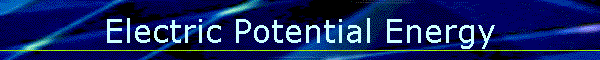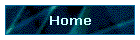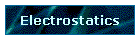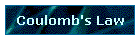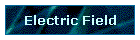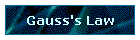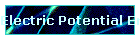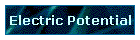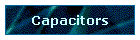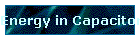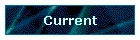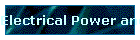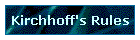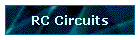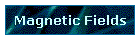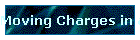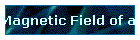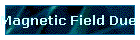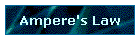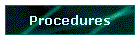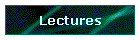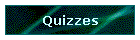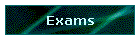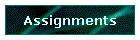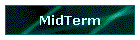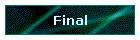Phys 4, Section 1                                                             Jan 25, 2002 Electric Potential Energy If forces act, then work can be done; if work is done there may be changes in kinetic energy and potential energy. Note that the electric force is conservative; only when we treat resistance in circuits will we have to worry about losses. W = ∫F•ds If the force is conservative, the work W can be expressed in terms of potential energy U : Wab = -ΔUab Also, note that for conservative forces, the total mechanical energy K + U is constant. Note that W is work done by the system, not work done on the system by external forces. For charge qo in a uniform field E moving a distance d in the direction of E: W = Fd = qoEd The change ΔU is -qoEd since W = -ΔU Electric Potential Energy of Two Point Charges F = kqqo/r2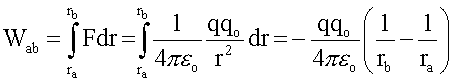Let ra be at infinity and define the potential energy between charges to be zero for infinite separation. Then for any charge qo located at distance r from charge q, the electric potential energy is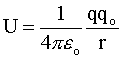Last modified on January 31, 2002